# Bifurcation analysis of fractional duffing system based on improved short memory principle method

## Ruiqun Ma1, Bo Zhang2, Jinglong Han3

1, 2, 3State Key Laboratory of Mechanics and Control of Mechanical Structures, Nanjing University of Aeronautics and Astronautics, Nanjing, 210016, China

3Corresponding author

Journal of Vibroengineering, Vol. 24, Issue 6, 2022, p. 1162-1173. https://doi.org/10.21595/jve.2022.22230
Received 29 September 2021; received in revised form 17 April 2022; accepted 29 April 2022; published 23 August 2022

Copyright © 2022 Ruiqun Ma, et al. This is an open access article distributed under the Creative Commons Attribution License, which permits unrestricted use, distribution, and reproduction in any medium, provided the original work is properly cited.
Views 28
WoS Core Citations 0
Abstract.

In this paper, the dynamic characteristics of fractional Duffing system are analyzed and studied by using the improved short memory principle method. This method has small amount of calculation and high precision, and can effectively improve the problem of large amount of calculation caused by the memory of fractional order. The influence of frequency change on the dynamic performance of the fractional Duffing system is studied using nonlinear dynamic analysis methods, such as Phase Portrait, Poincare Map and Bifurcation Diagram. Moreover, the dynamic behaviour of the fractional Duffing system when the fractional order and excitation amplitude changes are investigated. The analysis shows that when the excitation frequency changes from 0.43 to 1.22, the bifurcation diagram contains four periodic and three chaotic motion regions. Periodic motion windows are found in the three chaotic motion regions. It is confirmed that the frequency and amplitude of the external excitation and the fractional order of damping have a greater impact on system dynamics. Thus, attention shall be paid to the design and analysis of system dynamics.

Keywords: fractional calculus, bifurcation, chaos, short memory principle.

#### 1. Introduction

Fractional calculus was proposed almost at the same time as integer calculus. Its development was relatively slow for nearly 300 years due to the lack of a clear physical meaning of fractional calculus, and it was mainly studied in the field of pure mathematics. In 1974, Oldham and Spanier  co-authored the first monograph on fractional calculus titled ‘Applied Fractional Calculus’, which paved its way towards the application of fractional calculus. In the 1970s, Mandelbrot  pointed out that many fractional dimensions exist in nature. Since then, the theory of fractional calculus developed rapidly, and numerous applications of fractional calculus emerged -. The application of fractional calculus in mechanical engineering gradually increased in recent years mainly because many physical objects had fractional characteristics, such as viscoelasticity, damping, mechanical friction and impact -.

Many popular fractional systems, such as the Lorenz system , the Rossler system , Chua’s circuit  and the Duffing system , were used for research. The Duffing system was applied in many fields, including fluid-induced vibration, large-amplitude oscillation of centrifugal speed control systems and mathematical modeling , , . The Duffing system that considers fractional differential damping is equivalent to introducing polymer damping into the classic Duffing system. Compared with the traditional integer-order damping model, the fractional-order damping model can more accurately describe the dynamic behavior of the system -. In Reference , the nonlinear dynamic behavior of the fractional-order Duffing system was studied using the Adams-Bashforth-Moulton predictor-corrector method. The Adomian decomposition method was utilized to find the numerical solution to the combined fractional differential equation of the Duffing equation and the Van der Pol equation in Reference . The Melnikov method transforms a fractional-order system into an equivalent integer-order system, which can effectively detect chaos in the fractional-order Duffing system . Based on the definition formula with an amount of calculations, reference  studies the bifurcation and vibration resonance of fractional double-damping Duffing time delay system driven by external excitation signal with two wildly different frequencies. In Ref. , phase diagrams, bifurcation diagrams and Lyapunov exponents are used to determine the presence of chaos over a wide range of fractional orders, based on the Caputo fractional difference form of Duffing maps.

In this study, the improved short memory principle method is used to study the dynamic characteristics of the fractional Duffing system. Firstly, the Grünwald-Letnikov definition of fractional calculus is adopted. Secondly, the classical short memory principle is improved, and the numerical solution of the system is obtained. Lastly, the influence of excitation frequency on the dynamic performance of the fractional Duffing system is studied using nonlinear dynamic analysis methods, such as phase portrait, Poincare map and bifurcation diagram. Moreover, the dynamic behavior of the fractional Duffing system when the external excitation amplitude and fractional order change are investigated.

#### 2.1. Fractional differential definition

The three most frequently used definitions for fractional derivatives are Grünwald-Letnikov, Riemman-Liouville and Caputo definitions. The Grünwald-Letnikov definition is used in this work, as it is expressed as:

(1)
${}_{{t}_{0}}{}^{GL}{D}_{t}^{\alpha }x\left(t\right)=\underset{h\to 0}{\mathit{lim}}\frac{1}{{h}^{\alpha }}{\sum }_{j=0}^{\left[\left(t-{t}_{0}\right)/h\right]}{\left(-1\right)}^{j}\left(\begin{array}{c}\alpha \\ j\end{array}\right)x,$

where $\alpha$ is the fractional order, $h$ is the time step and [∙] means rounding. If the time step $h$ is small enough, the above-mentioned formula can be written as:

(2)
${}_{{t}_{0}}{}^{GL}{D}_{t}^{\alpha }x\left(t\right)\approx \frac{1}{{h}^{\alpha }}{\sum }_{j=0}^{\left[\left(t-{t}_{0}\right)/h\right]}{w}_{j}x\left(t-jh\right),$

where ${w}_{j}$ is the coefficient of binomial ${\left(1-z\right)}^{\alpha }$:

(3)
${w}_{j}={\left(-1\right)}^{j}\left(\begin{array}{c}\alpha \\ j\end{array}\right).$

Eq. (3) is changed to the recursive form:

(4)
${w}_{j}=\left(1-\frac{\alpha +1}{j}\right){w}_{j-1}.$

Its first item ${w}_{0}=1$.

#### 2.2. Improved short memory principle method

The classical short memory principle truncates the memory time. In Eq. (2), the upper limit of summation becomes $\left[L/h\right]$, where $L$ is the fixed memory time length, and the part exceeding $L$ is discarded. The discarded part often brings errors that cannot be ignored. In particular, when fractional order $\alpha$ approaches zero, the binomial coefficient changes slowly.

The improved short memory principle method changes the truncation of the memory time in the classic short memory principle to the number of terms of the binomial coefficient. Then, the finite binomial coefficient is repeatedly applied to gradually enlarging time steps for error reduction. The expression of a time step being enlarged for the first time is:

(5)
${D}_{t}^{\alpha }x\left(t\right)=\frac{1}{{h}^{\alpha }}{\sum }_{j=0}^{N}{w}_{j}x\left(t-jh\right)+\frac{1}{{\left(mh\right)}^{\alpha }}{\sum }_{j=\left[\frac{N}{m}\right]+1}^{\left[t/\left(mh\right)\right]}{w}_{j}x\left(t-jmh\right).$

The definition of Grünwald-Letnikov is used in this study, and the formulas are no longer marked. In Eq. (5), $N$ is the number of binomial truncated terms and $m$ is the magnification of the step length. Similarly, the expression of the second enlargement of step size is:

(6)
${D}_{t}^{\alpha }x\left(t\right)=\frac{1}{{h}^{\alpha }}{\sum }_{j=0}^{N}{w}_{j}x\left(t-jh\right)+\frac{1}{{\left(mh\right)}^{\alpha }}{\sum }_{j=\left[\frac{N}{m}\right]+1}^{N}{w}_{j}x\left(t-jmh\right)$

In the same manner, other expressions of step size can be derived.

#### 2.3. Basis for step enlargement

Step enlargement is based on the initial time step. After the step size is enlarged, although the interval for taking the function value increases, the binomial coefficient of the function value also increases, and the magnification is almost the same as the step size magnification. For example, Eq. (5) can be rewritten as:

(7)
${D}_{t}^{\alpha }x\left(t\right)=\frac{1}{{h}^{\alpha }}\left(\sum _{j=0}^{N}{w}_{j}x\left(t-jh\right)+\sum _{j=\left[\frac{N}{m}\right]+1}^{\left[t/\left(mh\right)\right]}\frac{{w}_{j}}{{m}^{\alpha }}x\left(t-jmh\right)\right).$

The second term at the right end of the formula above is the result of the step size being enlarged for the first time. The binomial coefficient before the function value $x\left(t-jmh\right)$ is ${w}_{j}/{m}^{\alpha }$. If the step size is not enlarged, the binomial coefficient in front of the function value shall be ${w}_{jm}$. Table 1 shows the ratio of the corresponding binomial coefficients when $N=$ 100, $\alpha =$ 0.5 and $m$ are 5, 10 and 20, respectively. $A$ is the ratio of binomial coefficient ${w}_{j}/{m}^{\alpha }$ after the first step is enlarged to binomial coefficient ${w}_{jm}$. $B$ is the ratio of binomial coefficient ${w}_{j}/{m}^{2\alpha }$ after the second step is enlarged to binomial coefficient ${w}_{j{m}^{2}}$. The ratio is almost equal to step magnification $m$ and ${m}^{2}$, and the ratio increases with the increase in step magnification. After this increase, the first binomial coefficient increases; in particular, when 20, it is larger than the number of initial steps in the enlarged step. A large magnification causes a large error. In addition, whether the adopted function value is representative in the interval, that is, whether the adopted function value is close to the average value of the function value in the interval, which is also a question to be considered. The error in the long-term calculation is limited because expanding the step size to derive the function value is a dynamic ergodic process. In summary, it is required to try to intercept as many binomial coefficients as possible if the calculation conditions permit, and a small step size magnification shall be selected to obtain a highly accurate numerical solution.

Table 1. Partial results of $\frac{{w}_{j}/{m}^{\alpha }}{{w}_{jm}}$ and $\frac{{w}_{j}/{m}^{2\alpha }}{{w}_{j{m}^{2}}}$ when $\alpha =$ 0.5

 $m=\text{5}$ $m=\text{10}$ $m=\text{20}$ $j$ $\frac{{w}_{j}/{m}^{\alpha }}{{w}_{jm}}$ $\frac{{w}_{j}/{m}^{2\alpha }}{{w}_{j{m}^{2}}}$ $j$ $\frac{{w}_{j}/{m}^{\alpha }}{{w}_{jm}}$ $\frac{{w}_{j}/{m}^{2\alpha }}{{w}_{j{m}^{2}}}$ $j$ $\frac{{w}_{j}/{m}^{\alpha }}{{w}_{jm}}$ $\frac{{w}_{j}/{m}^{2\alpha }}{{w}_{j{m}^{2}}}$ 6 21.302 427.31 11 10.3225 103.543 11 20.6804 414.279 21 5.07335 25.4396 21 10.1649 101.813 21 20.348 407.306 31 5.04926 25.2953 31 10.1108 101.218 31 20.2338 404.909 41 5.03708 25.2224 41 10.0834 100.917 41 20.176 403.696 51 5.02973 25.1783 51 10.0669 100.735 51 20.1412 402.964 61 5.02481 25.1488 61 10.0558 100.614 61 20.1178 402.474 71 5.02129 25.1277 71 10.0479 100.527 71 20.1011 402.123 81 5.01865 25.1118 81 10.0419 100.461 81 20.0885 401.859 91 5.01658 25.0995 91 10.0373 100.41 91 20.0788 401.654 100 5.01508 25.0905 100 10.0339 100.373 100 20.0716 401.504

#### 2.4. Fractional duffing system

The form of the classic integer-order Duffing system is:

(8)

where $D$ is the first-order differential operator, ${D}^{2}$ is the second-order differential operator, $m$ is the mass, $c$ is the damping coefficient, $k$ is the linear stiffness coefficient, $a$ is the nonlinear stiffness coefficient and $f$ and $\omega$ are the amplitude and frequency of external excitation, respectively. If the damping term is changed to the fractional order, it becomes a fractional-order Duffing system:

(9)
$m{D}^{2}x\left(t\right)+c{D}^{\alpha }x\left(t\right)+kx\left(t\right)+a{x}^{3}\left(t\right)=f\mathrm{sin}\left(\omega t\right),$

where $\alpha$ is the fractional order of the damping of the fractional Duffing system. In this study, the improved short memory principle is used to solve the fractional Duffing equation.

#### 3. Nonlinear dynamic analysis of fractional duffing system

In this section, several fixed parameters in Eq. (9) are taken as $m=$ 1, $c=$ 0.9, $k=$ –1 and $a=$ 1. The initial conditions are $x\left(0\right)=0$ and $\stackrel{˙}{x}\left(0\right)=0$.

#### 3.1. Fractional order duffing system with $\mathbit{\alpha }=1.2$ and $\mathbit{f}=0.6$

Fractional order $\alpha =$ 1.2 and excitation amplitude $f=$ 0.6 are adopted to analyze the influence of excitation frequency on the system. The calculation parameters are as follows: the basic step size is $h=$ 0.001, the number of truncated items is $Nt=$ 10,000, and the step length magnification is $m=$ 5. Excitation frequency $\omega$ is changed from 0.43 to 1.23 in the experiment to analyze the influence of excitation frequency on the fractional Duffing system, and the change step is 0.001. A total of 250 cycles is calculated for each frequency point, and the first 150 cycles are discarded. The bifurcation diagram of the system obtained with $\omega$ as the control parameter is shown in Fig. 1. The figure indicates that excitation frequency $\omega$ has a relatively large impact on the dynamic behavior of the system. Three chaotic and four periodic motion regions exist during the change of $\omega$ from 0.43 to 1.22.

The system performance in response to stable period-1 motion occurs at $0.43<\omega \le 0.487$. The phase portrait and Poincaré map when $\omega =$ 0.45 are shown in Figs. 2(a1) and 2(a2), respectively. The phase portrait is a universal limit cycle; correspondingly, the Poincaré map exhibits an isolated point. Notably, $0.488<\omega \le 0.518$ is the first chaotic motion region of the system.

Fig. 1. Bifurcation diagram of fractional-order Duffing system with $\alpha =$ 1.2, $0.43<\omega \le 1.23$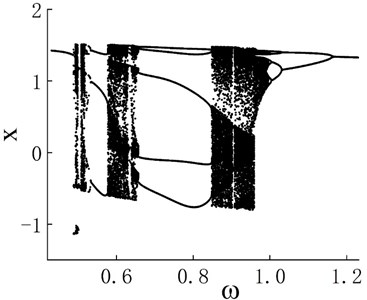Fig. 2. Phase portraits and Poincaré maps of fractional Duffing system. a1) Phase portrait with $\omega =\text{0.45}$, a2) Poincaré map with $\omega =\text{0.45}$, b1) phase portrait with $\omega =\text{0.509}$, b2) Poincaré map with $\omega =\text{0.509}$, c1) phase portrait with $\omega =\text{0.55}$, c2) Poincaré map with $\omega =\text{0.55}$, d1) phase portrait with $\omega =\text{0.6}$, d2) Poincaré map with $\omega =\text{0.6}$, e1) phase portrait with $\omega =\text{0.8}$, e2) Poincaré map with $\omega =\text{0.8}$, f1) phase portrait with , f2) Poincaré map with $\omega =\text{0.88}$, g1) phase portrait with $\omega =\text{1.02}$ and g2) Poincaré map with $\omega =\text{1.02}$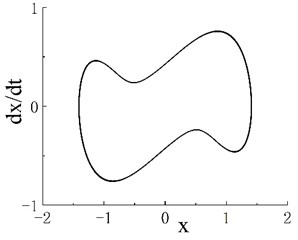a1)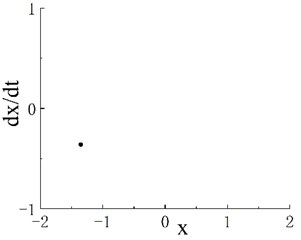a2)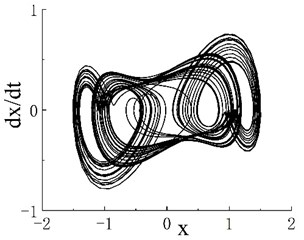b1)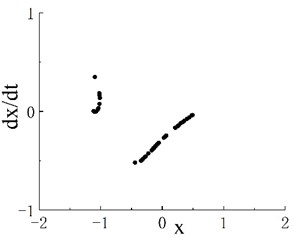b2)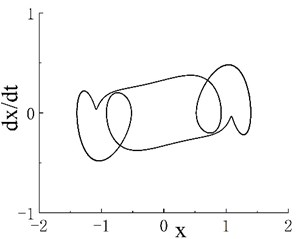c1)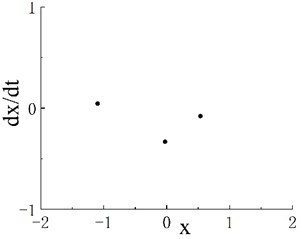c2)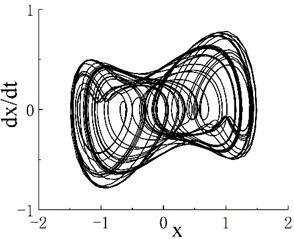d1)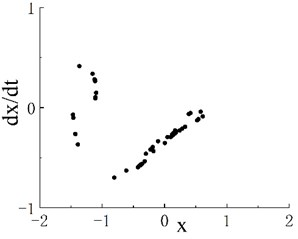d2)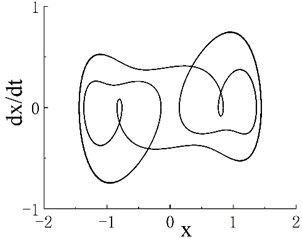e1)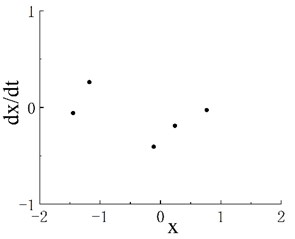e2)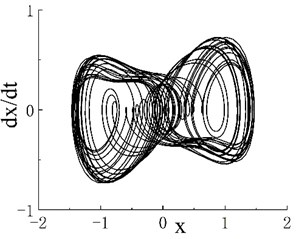f1)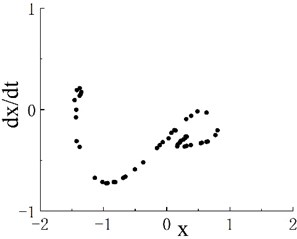f2)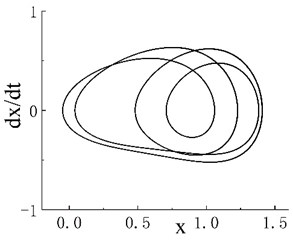g1)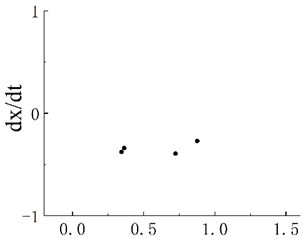g2)

The phase portrait and Poincaré map when $\omega =\text{0.509}$ are shown in Figs. 2(b1) and 2(b2), respectively. On the Poincaré map, many discrete points are concentrated in two areas. With the increase in excitation frequency $\omega$, the system enters the period-3 motion region, and its frequency range is $0.519\le \omega \le 0.577$. The phase portrait and Poincaré map when $\omega =$ 0.55 are shown in Figs. 2(c1) and 2(c2), respectively. The Poincaré map appears as three isolated points, and $0.578\le \omega \le 0.657$ is the second chaotic region of the system. The phase portrait when $\omega =$ 0.6 is shown as Fig. 2(d1), and scattered points can be seen on the corresponding Poincaré map in Fig. 2(d2). When $\omega \ge 0.658$, the system enters a stable period-5 motion region, and its interval is $0.658\le \omega \le 0.848$. The phase portrait and Poincaré map when $\omega =$ 0.8 are shown in Figs. 2(e1) and 2(e2), respectively. The Poincaré map has five scattered points, and $0.849\le \omega \le 0.995$ is the third chaotic motion region. Fig. 2(f1) shows the phase portrait when $\omega =$ 0.88, and the corresponding Poincaré map is given in Fig. 2(f2). As $\omega$ further increases, the system enters periodic motion from chaotic motion. Fig. 1 indicates that when $\omega \ge$ 1.17, the system moves stably in period 1. A period-doubling bifurcation transition region exists between the periodic motion region and the third chaotic motion region. The phase portrait and Poincaré map of the transition region when $\omega =$ 1.02 are shown in Figs. 2(g1) and 2(g2), respectively.

Notably, periodic motion windows are present in the three chaotic motion regions. The bifurcation diagram of the periodic motion window in the first chaotic motion region is shown in Fig. 3(a). The chaotic motion transits from period-doubling motion to period-2 motion. The phase portrait and Poincaré map when $\omega =$ 0.506 in the period window are shown in Figs. 3(b) and 3(c), respectively. The bifurcation diagram of the periodic motion window in the second chaotic motion region is shown in Fig. 4(a). In the period window, $\omega =$ 0.635 is period-4 motion, and its phase portrait and Poincaré map are shown in Figs. 3(b) and 3(c), respectively. Fig. 5(a) presents the bifurcation diagram of the periodic window in the third chaotic region. Figs. 5(b) and 5(c) respectively show the phase portrait and corresponding Poincaré map when $\omega =$ 0.904 in the window.

Fig. 3. a) Bifurcation diagram of periodic window in chaotic motion region 1, b) phase portrait when $\omega =$ 0.506 and c) Poincaré map when $\omega =$ 0.506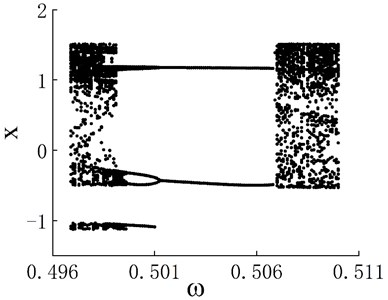a)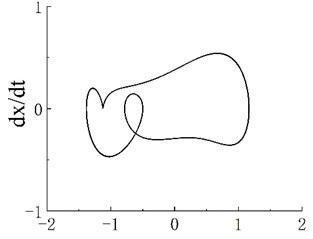b)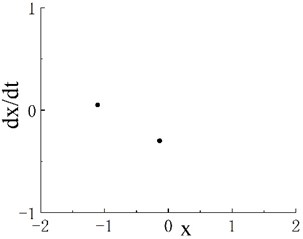c)

Fig. 4. a) Bifurcation diagram of periodic window in chaotic motion region 2, b) phase portrait when $\omega =$ 0.635 and c) Poincaré map when $\omega =$ 0.635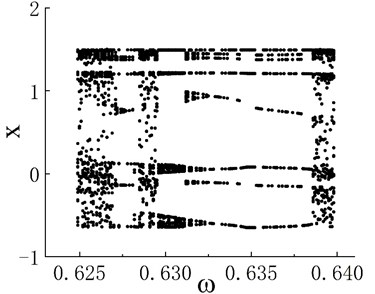a)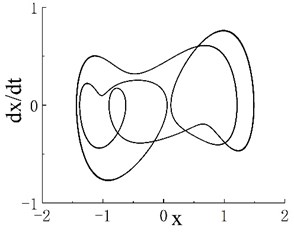b)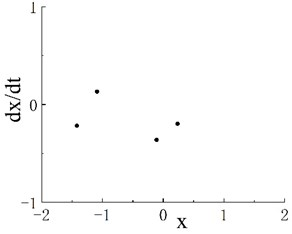c)

Fig. 5. a) Bifurcation diagram of periodic window in chaotic motion region 3, b) phase portrait when $\omega =$ 0.904 and c) Poincaré map when $\omega =$ 0.904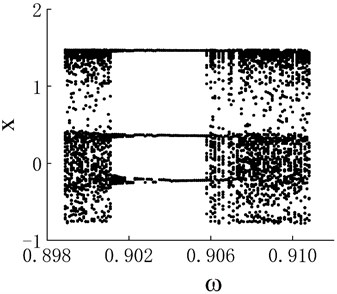a)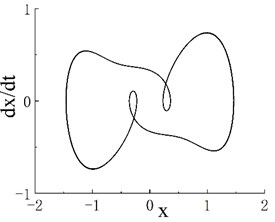b)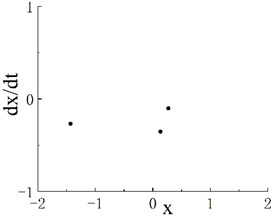c)

Notably, an interesting change occurs in the periodic window of the second chaotic motion region. Although the system moves in period 4 of the window, the bifurcation diagram has eight dotted lines instead of four solid lines. Although the system exhibits period-4 motion in the period window, the bifurcation diagram has eight dotted lines instead of four solid lines. This observation shows that the motion of the system jumps with the change in frequency in the period window, but it is still period-4 motion. For example, the phase portraits when $\omega =$ 0.6352 and $\omega =$ 0.6353 are shown in Figs. 6(a) and 6(b), respectively. The phase portraits of two adjacent frequency points are rotated by exactly 180 degrees. When the frequency is further refined, this jumping property remains. Figs. 6(c) and 6(d) present phase portraits with $\omega$ of 0.63280 and 0.63281, respectively.

Fig. 6. a) Phase portrait with $\omega =$ 0.6352, b) phase portrait with $\omega =$ 0.6353, c) phase portrait with $\omega =$ 0.63280 and d) phase portrait with $\omega =$ 0.63281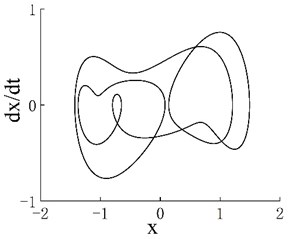a)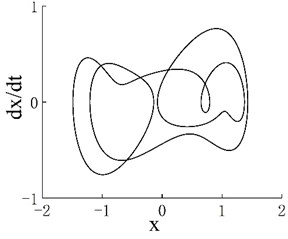b)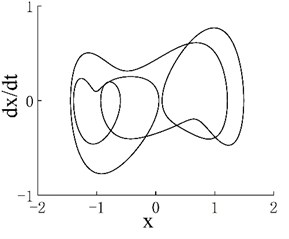c)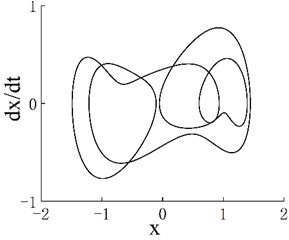d)

#### 3.2. Fractional order duffing system with fractional order $\mathbit{\alpha }$ or excitation amplitude $\mathbit{f}$ fluctuations

This paper also examines the dynamic characteristics of the system when the fractional order changes. The excitation amplitude is $f=$ 1, the excitation frequency is $\omega =$ 1, the basic step length is $h=$ 0.001, the basic truncation term is $Nt=$ 10,000, and the step length magnification is $m=$ 5. its memory weakens as the fractional order increases. Thus, the number of truncated items ${N}_{t}$ is set to decrease by 1000 items with each increase in $\alpha$ by 0.2. The bifurcation diagram is shown in Fig. 7(a). In the process of changing fractional order $\alpha$ from 0.2 to 2, three periodic and three chaotic motion regions appear. The transition is from the first chaotic zone to a stable period-1 motion region after period-doubling bifurcation. As $\alpha$ continues to increase, it enters the second chaotic motion region through period-doubling bifurcation.

Fig. 7(b) presents the bifurcation diagram of the system when excitation amplitude $f$ fluctuates from 0.1 to 2.1. The fractional order is $\alpha =$ 0.8, the excitation frequency is $\omega =$ 1, the basic step length is $h=$ 0.001, the basic truncation item number is $Nt=$ 10,000, and the step length magnification is $m=$ 5. The figure shows that three periodic and two chaotic motion regions appear during the fluctuation of excitation amplitude. The first is a stable period-1 motion; the first chaotic motion region is entered through period-doubling bifurcation, followed by entrance to the period-3 motion region. A part of the period-6 movement is contained in the period-3 motion region, and the second chaotic motion region is entered from period-3 motion. As the excitation amplitude continues to increase, the system transitions from the second chaotic region to the period-1 motion region through the period-doubling bifurcation.

Fig. 7. a) Bifurcation diagram of $x$ versus $\alpha$, $0.2<\alpha <2$ and b) bifurcation diagram of $x$ versus $f$, $0.1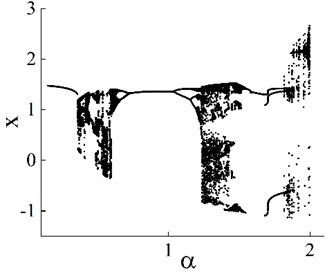a)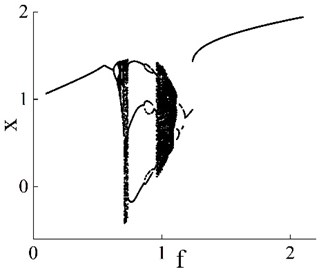b)

#### 4. Conclusions

An improved short memory principle method is introduced to solve the fractional Duffing system numerically. And based on high computational efficiency, some previously unobserved phenomena are obtained. The influence of excitation frequency on the dynamic performance of the system is studied using nonlinear dynamic analysis methods, such as phase portrait, Poincaré map and bifurcation diagram. The bifurcation diagram has four periodic and three chaotic motion regions. The third chaotic motion region exhibits an obvious transition from period-doubling motion to stable period-1 motion. In addition, periodic motion windows are found in the three chaotic motion regions. The periodic motion of the periodic window in the second chaotic motion region has a jumping property. The influence of excitation amplitude and fractional order on the dynamic system performance is also studied. The results show that a change in the system parameters of the fractional Duffing equation affects the dynamic system performance. Thus, such a change shall be considered during the dynamic system design and analysis.

#### Acknowledgements

This work was supported by the National Natural Science Foundation of China [Grant No. 11472133].

1. K. B. Oldham and J. Spanier, The Fractional Calculus: Theory and Applications of Differentiation and Integration to Arbitrary Order. Dover Publications, 1974. [Search CrossRef]
2. Cannon J. W. and Mandelbrot B. B., “The Fractal Geometry of Nature,” The American Mathematical Monthly, 1984. [Publisher]
3. R. L. Bagley and P. J. Torvik, “Fractional calculus in the transient analysis of viscoelastically damped structures,” AIAA Journal, Vol. 23, No. 6, pp. 918–925, Jun. 1985, https://doi.org/10.2514/3.9007 [Publisher]
4. R. L. Bagley and P. J. Torvik, “Fractional calculus – A different approach to the analysis of viscoelastically damped structures,” AIAA Journal, Vol. 21, No. 5, pp. 741–748, May 1983, https://doi.org/10.2514/3.8142 [Publisher]
5. Y. Yu, H.-X. Li, S. Wang, and J. Yu, “Dynamic analysis of a fractional-order Lorenz chaotic system,” Chaos, Solitons and Fractals, Vol. 42, No. 2, pp. 1181–1189, Oct. 2009, https://doi.org/10.1016/j.chaos.2009.03.016 [Publisher]
6. T. T. Hartley, C. F. Lorenzo, and H. Killory Qammer, “Chaos in a fractional order Chua’s system,” IEEE Transactions on Circuits and Systems I: Fundamental Theory and Applications, Vol. 42, No. 8, pp. 485–490, 1995, https://doi.org/10.1109/81.404062 [Publisher]
7. O. P. Agrawal, “A general formulation and solution scheme for fractional optimal control problems,” Nonlinear Dynamics, Vol. 38, No. 1-4, pp. 323–337, Dec. 2004, https://doi.org/10.1007/s11071-004-3764-6 [Publisher]
8. Y. Chen, B. M. Vinagre, and I. Podlubny, “Continued fraction expansion approaches to discretizing fractional order derivatives – an expository review,” Nonlinear Dynamics, Vol. 38, No. 1-4, pp. 155–170, Dec. 2004, https://doi.org/10.1007/s11071-004-3752-x [Publisher]
9. S. Jiménez, J. A. González, and L. Vázquez, “Fractional Duffing’s equation and geometrical resonance,” International Journal of Bifurcation and Chaos, Vol. 23, No. 5, p. 1350089, May 2013, https://doi.org/10.1142/s0218127413500892 [Publisher]
10. J.-H. Jia, X.-Y. Shen, and H.-X. Hua, “Viscoelastic behavior analysis and application of the fractional derivative maxwell model,” Journal of Vibration and Control, Vol. 13, No. 4, pp. 385–401, Apr. 2007, https://doi.org/10.1177/1077546307076284 [Publisher]
11. A. Shokooh and L. Suárez, “A comparison of numerical methods applied to a fractional model of damping materials,” Journal of Vibration and Control, Vol. 5, No. 3, pp. 331–354, May 1999, https://doi.org/10.1177/107754639900500301 [Publisher]
12. R. S. Barbosa and J. A. T. Machado, “Describing function analysis of systems with impacts and backlash,” Nonlinear Dynamics, Vol. 29, No. 1/4, pp. 235–250, 2002, https://doi.org/10.1023/a:1016514000260 [Publisher]
13. W. Zhang, S. Zhou, H. Li, and H. Zhu, “Chaos in a fractional-order Rössler system,” Chaos, Solitons and Fractals, Vol. 42, No. 3, pp. 1684–1691, Nov. 2009, https://doi.org/10.1016/j.chaos.2009.03.069 [Publisher]
14. J. A. T. Machado, D. Baleanu, W. Chen, and J. Sabatier, “New trends in fractional dynamics,” Journal of Vibration and Control, Vol. 20, No. 7, pp. 963–963, May 2014, https://doi.org/10.1177/1077546313507652 [Publisher]
15. X. Li and R. Wu, “Hopf bifurcation analysis of a new commensurate fractional-order hyperchaotic system,” Nonlinear Dynamics, Vol. 78, No. 1, pp. 279–288, Oct. 2014, https://doi.org/10.1007/s11071-014-1439-5 [Publisher]
16. D. Chen, C. Wu, H. H. C. Iu, and X. Ma, “Circuit simulation for synchronization of a fractional-order and integer-order chaotic system,” Nonlinear Dynamics, Vol. 73, No. 3, pp. 1671–1686, Aug. 2013, https://doi.org/10.1007/s11071-013-0894-8 [Publisher]
17. Y.-J. Shen, P. Wei, and S.-P. Yang, “Primary resonance of fractional-order van der Pol oscillator,” Nonlinear Dynamics, Vol. 77, No. 4, pp. 1629–1642, Sep. 2014, https://doi.org/10.1007/s11071-014-1405-2 [Publisher]
18. N. Srinil and H. Zanganeh, “Modelling of coupled cross-flow/in-line vortex-induced vibrations using double Duffing and van der Pol oscillators,” Ocean Engineering, Vol. 53, pp. 83–97, Oct. 2012, https://doi.org/10.1016/j.oceaneng.2012.06.025 [Publisher]
19. P. Brzeski, P. Perlikowski, and T. Kapitaniak, “Numerical optimization of tuned mass absorbers attached to strongly nonlinear Duffing oscillator,” Communications in Nonlinear Science and Numerical Simulation, Vol. 19, No. 1, pp. 298–310, Jan. 2014, https://doi.org/10.1016/j.cnsns.2013.06.001 [Publisher]
20. N. M. M. Maia, J. M. M. Silva, and A. M. R. Ribeiro, “On a general model for damping,” Journal of Sound and Vibration, Vol. 218, No. 5, pp. 749–767, Dec. 1998, https://doi.org/10.1006/jsvi.1998.1863 [Publisher]
21. T. T. Hartley and C. F. Lorenzo, “A frequency-domain approach to optimal fractional-order damping,” Nonlinear Dynamics, Vol. 38, No. 1-4, pp. 69–84, Dec. 2004, https://doi.org/10.1007/s11071-004-3747-7 [Publisher]
22. Y. Wang and J.-Y. An, “Amplitude-frequency relationship to a fractional Duffing oscillator arising in microphysics and tsunami motion,” Journal of Low Frequency Noise, Vibration and Active Control, Vol. 38, No. 3-4, pp. 1008–1012, Dec. 2019, https://doi.org/10.1177/1461348418795813 [Publisher]
23. Z. Li, D. Chen, J. Zhu, and Y. Liu, “Nonlinear dynamics of fractional order Duffing system,” Chaos, Solitons and Fractals, Vol. 81, pp. 111–116, Dec. 2015, https://doi.org/10.1016/j.chaos.2015.09.012 [Publisher]
24. Duan Junsheng, Sun Jie, and Temuer Chaolu, “Nonlinear fractional differential equation combining duffing equation and van der pol equation,” Shuxue Zazhi, Vol. 31, No. 1, pp. 7–10, 2011. [Search CrossRef]
25. J. Niu, R. Liu, Y. Shen, and S. Yang, “Chaos detection of Duffing system with fractional-order derivative by Melnikov method,” Chaos: An Interdisciplinary Journal of Nonlinear Science, Vol. 29, No. 12, p. 123106, Dec. 2019, https://doi.org/10.1063/1.5124367 [Publisher]
26. R. Wang, H. Zhang, and Y. Zhang, “Bifurcation and vibration resonance in the time delay Duffing system with fractional internal and external damping,” Meccanica, Vol. 57, No. 5, pp. 999–1015, May 2022, https://doi.org/10.1007/s11012-022-01483-y [Publisher]
27. A. Ouannas, A.-A. Khennaoui, S. Momani, and V.-T. Pham, “The discrete fractional duffing system: Chaos, 0-1 test, C0 complexity, entropy, and control,” Chaos: An Interdisciplinary Journal of Nonlinear Science, Vol. 30, No. 8, p. 083131, Aug. 2020, https://doi.org/10.1063/5.0005059 [Publisher]

#### Cited By

 Mathematics Valentine Aleksandrovich Kim, Roman Ivanovich Parovik, Zafar Ravshanovich Rakhmonov 2023